Ex 4.4

Chapter 4 Class 12 Determinants
Serial order wise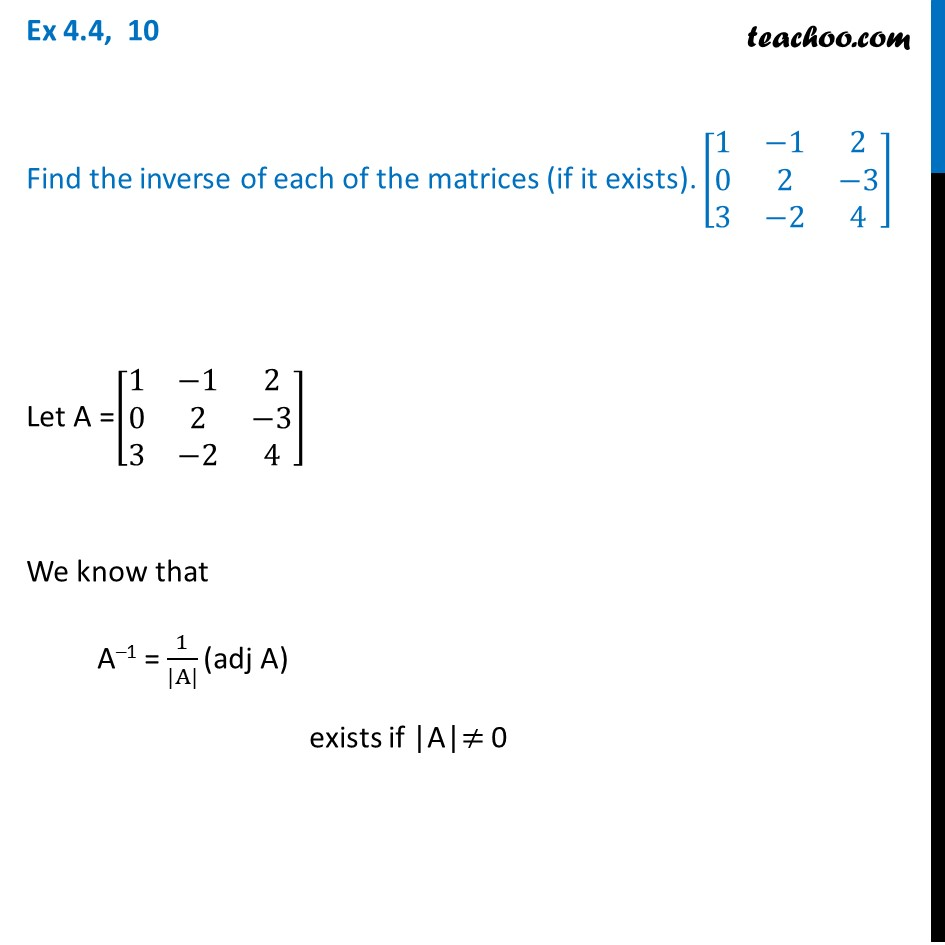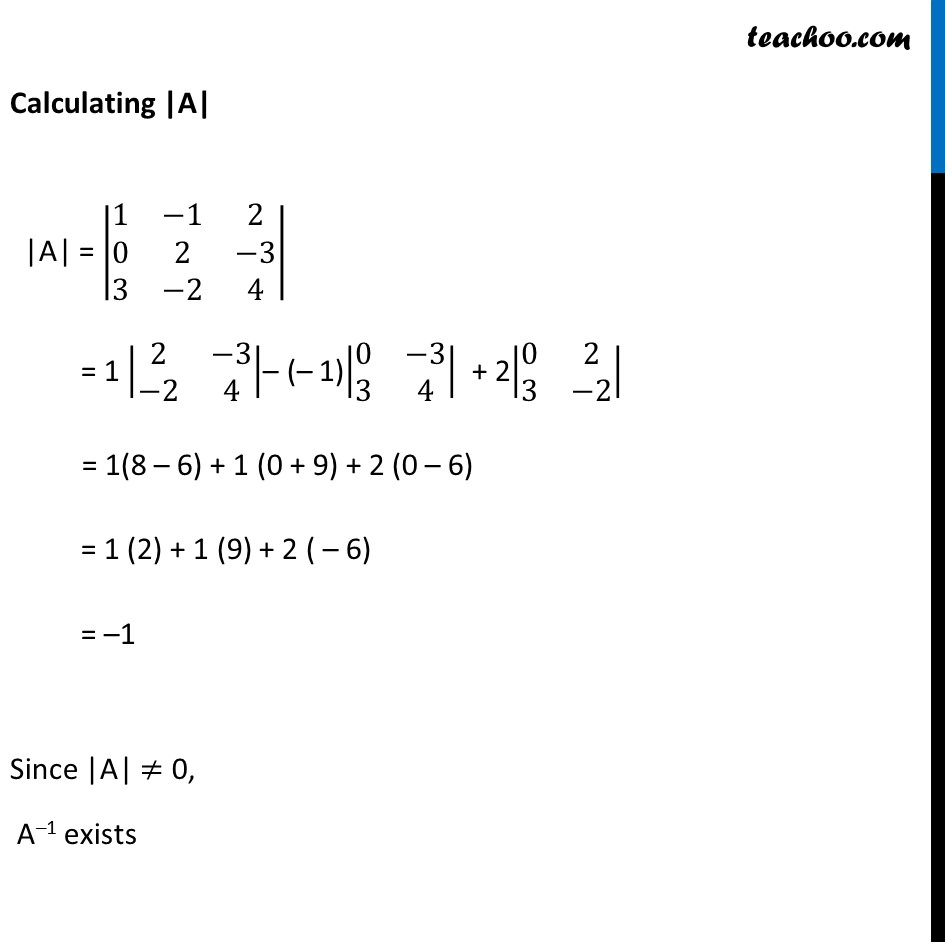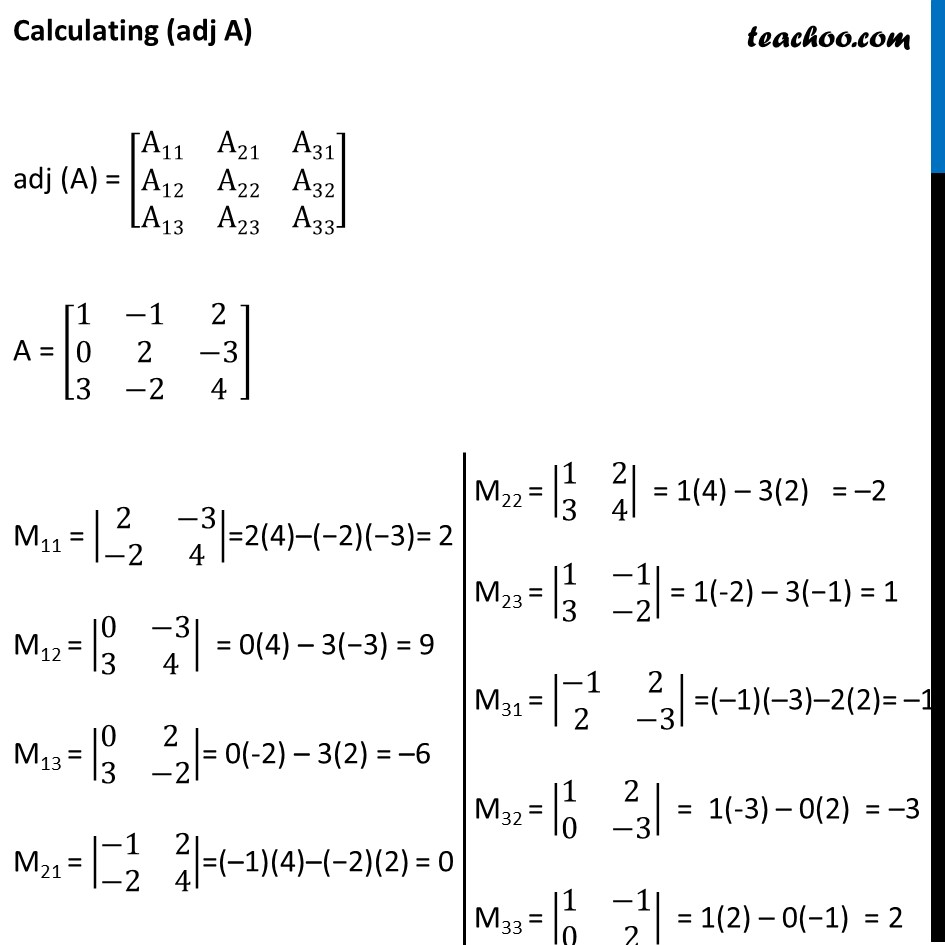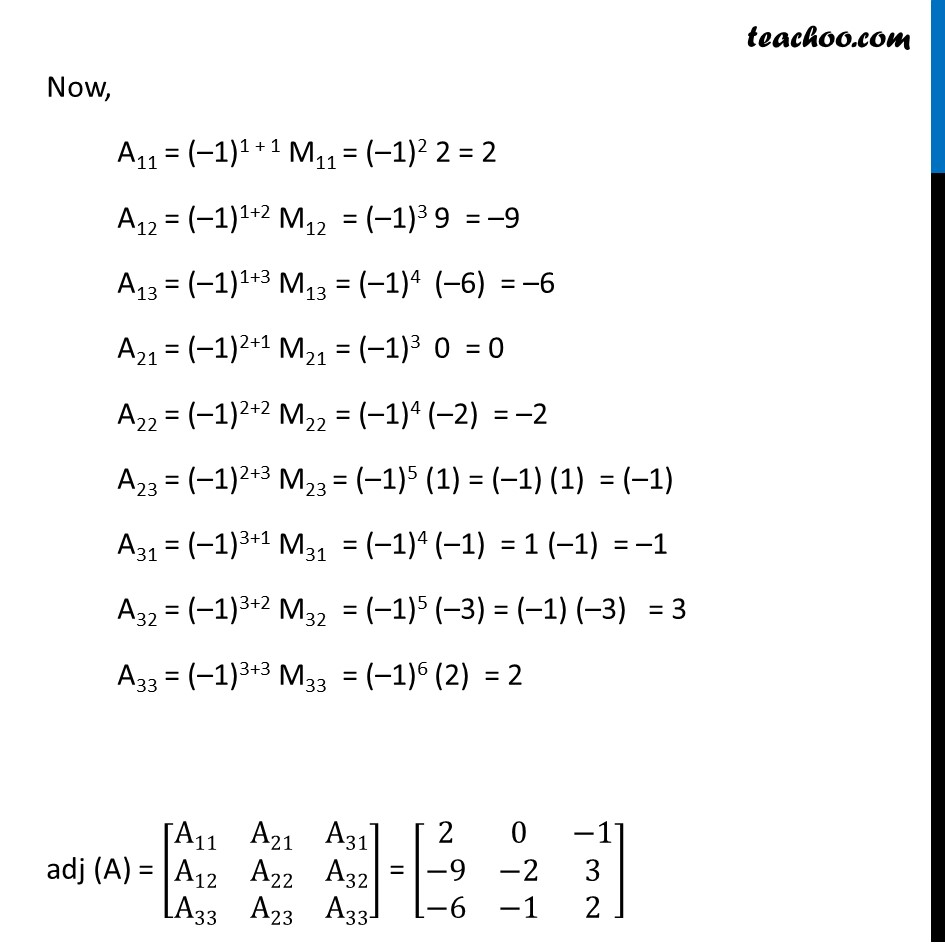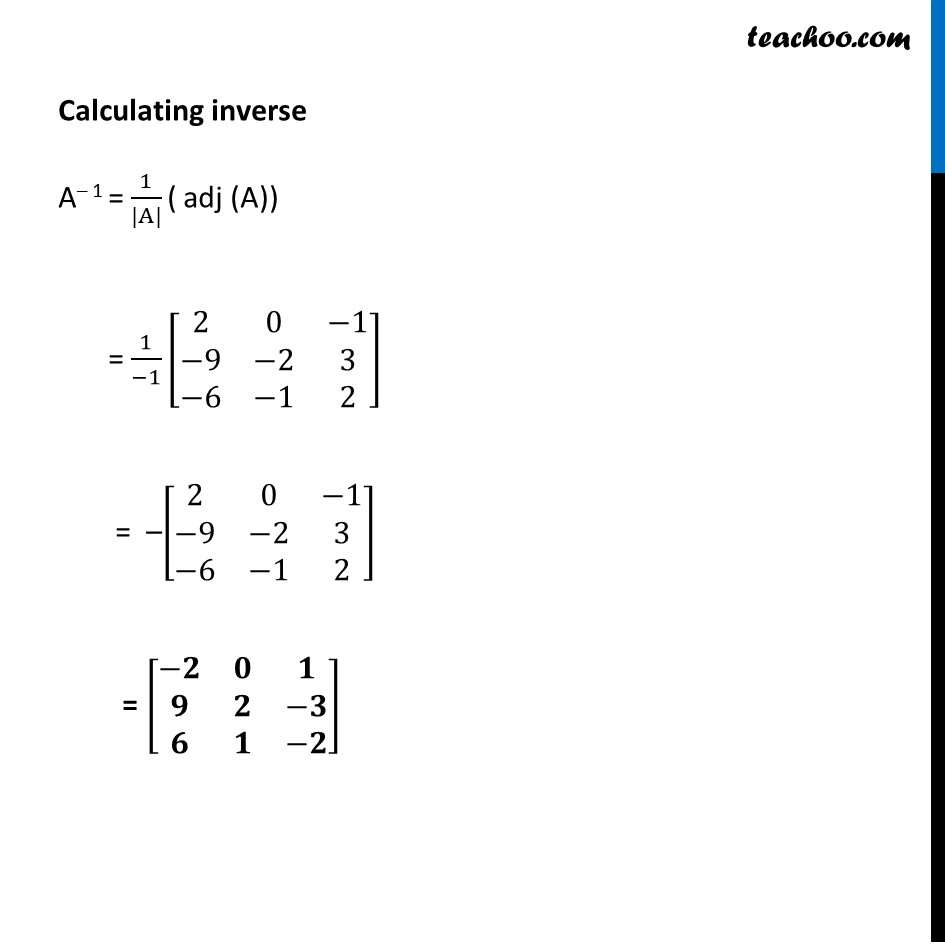Learn in your speed, with individual attention - Teachoo Maths 1-on-1 Class

### Transcript

Ex 4.4, 10 Find the inverse of each of the matrices (if it exists). [■8(1&−1&2@0&2&−3@3&−2&4)] Let A =[■8(1&−1&2@0&2&−3@3&−2&4)] We know that A–1 = 1/(|A|) (adj A) exists if |A|≠ 0 Calculating |A| |A| = |■8(1&−1&2@0&2&−3@3&−2&4)| = 1 |■8(2&−3@−2&4)|– (– 1)|■8(0&−3@3&4)| + 2|■8(0&2@3&−2)| = 1(8 – 6) + 1 (0 + 9) + 2 (0 – 6) = 1 (2) + 1 (9) + 2 ( – 6) = –1 Since |A| ≠ 0, A–1 exists Calculating (adj A) adj (A) = [■8(A11&A21&A31@A12&A22&A32@A13&A23&A33)] A = [■8(1&−1&2@0&2&−3@3&−2&4)] M11 = |■8(2&−3@−2&4)|=2(4)–(−2)(−3)= 2 M12 = |■8(0&−3@3&4)| = 0(4) – 3(−3) = 9 M13 = |■8(0&2@3&−2)|= 0(-2) – 3(2) = –6 M21 = |■8(−1&2@−2&4)|=(–1)(4)–(−2)(2) = 0 M22 = |■8(1&2@3&4)| = 1(4) – 3(2) = –2 M23 = |■8(1&−1@3&−2)| = 1(-2) – 3(−1) = 1 M31 = |■8(−1&2@2&−3)| =(–1)(–3)–2(2)= –1 M32 = |■8(1&2@0&−3)| = 1(-3) – 0(2) = –3 M33 = |■8(1&−1@0&2)| = 1(2) – 0(−1) = 2 Now, A11 = (–1)1 + 1 M11 = (–1)2 2 = 2 A12 = (–1)1+2 M12 = (–1)3 9 = –9 A13 = (–1)1+3 M13 = (–1)4 (–6) = –6 A21 = (–1)2+1 M21 = (–1)3 0 = 0 A22 = (–1)2+2 M22 = (–1)4 (–2) = –2 A23 = (–1)2+3 M23 = (–1)5 (1) = (–1) (1) = (–1) A31 = (–1)3+1 M31 = (–1)4 (–1) = 1 (–1) = –1 A32 = (–1)3+2 M32 = (–1)5 (–3) = (–1) (–3) = 3 A33 = (–1)3+3 M33 = (–1)6 (2) = 2 adj (A) = [■8(A11&A21&A31@A12&A22&A32@A33&A23&A33)] = [■8(2&0&−1@−9&−2&3@−6&−1&2)] Calculating inverse A– 1 = 1/(|A|) ( adj (A)) = 1/(−1) [■8(2&0&−1@−9&−2&3@−6&−1&2)] = –[■8(2&0&−1@−9&−2&3@−6&−1&2)] = [■8(−𝟐&𝟎&𝟏@𝟗&𝟐&−𝟑@𝟔&𝟏&−𝟐)]